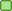###Author Topic: Object3D rotation around another object  (Read 3382 times)

####EgonOlsen

••• Posts: 12087##### Re: Object3D rotation around another object
« Reply #15 on: September 15, 2013, 08:57:28 pm »
You are still ignoring that translations are cumulative. Maybe your orbitCenter is so close to the origin that it doesn't matter, but it's not correct anyway. You are trying to calculate and absolute position in each iteration and you should treat it like one...but you don't...

####phmenard

• byte
•• Posts: 8##### [Solved]
« Reply #16 on: September 15, 2013, 11:29:10 pm »
I figured it out ... seems the + and - signs make a big difference when orbiting on the z axis ... so with that said here is the code if you want to make your own unique orbits ...

Code: [Select]
`myOrbitAngle += myOrbitSpeed; if(myOrbitAngle > Math.PI * 2)   myOrbitAngle %= Math.PI * 2;     SimpleVector sv = new SimpleVector();   //double rad = (myOrbitAngle * (Math.PI / 180)); // Converting Degrees To Radians   double x = myOrbit.getTransformedCenter().x - distanceFromParent * (Math.cos(myOrbitAngle)* .5);    double z = myOrbit.getTransformedCenter().z - distanceFromParent * (Math.sin(myOrbitAngle) * 1);           sv.x = (float) x;   sv.z = (float) z;   sv.y = 0;    myObject.clearTranslation();   myObject.translate(sv);   myObject.rotateY(myOrbitSpeed);`
if you want to orbit on a different axis just change around the x,y,z cords as needed ... as I said this code orbits the z axis. The floating point and whole number after the cos and sin add the option of playing around with the orbit ... stretch it to elliptical ... widen it ect.

Have fun ... I know I am ... now if I could only get a blazing sun rather than a dull object giving off light :-)